# To graph : The quadratic equation y = 8 x − x 2 in a graphing window.### Precalculus: Mathematics for Calcu...

6th Edition
Stewart + 5 others
Publisher: Cengage Learning
ISBN: 9780840068071### Precalculus: Mathematics for Calcu...

6th Edition
Stewart + 5 others
Publisher: Cengage Learning
ISBN: 9780840068071

#### Solutions

Chapter 1.9, Problem 10E
To determine

## To graph: The quadratic equation y=8x−x2 in a graphing window.

Expert Solution

### Explanation of Solution

Given information:

Graph:

The graph of the quadratic equation y=8xx2 can be sketched in the cartesian plane,

Consider the quadratic equation, y=8xx2 .

Rewrite the given equation by square both sides:

y=8xx2y2=8xx2 ,

Add both side of the equation 42 to make a square of two functions, (a+b)2 .

y2=8xx2y2+x28x=0y2+x2+428x=42y2+(x4)2=42

As the observation says this equation is an semicircle equation.

y always positive, roots given always positive value.

Either x4=0 or y0=0 :

Hence x=4 and y=0 .

Steps to plot the graph of the equation y=8xx2 with the help of graphing utility are as follows:

Step 1: Press MODE key.

Step 2: Use the down arrow key to reach FUNC option.

Step 3: Press ENTER key.

Step 4: Press Y= key.

Step 5: Enter the function y=8xx2 .

Step 6: Press WINDOW key. Change the settings to

Xmin=10Xmax=10Ymin=10Ymax=10

For better view of graph.

Step 8: Press GRAPH key.

The result obtained on the screen is provided below,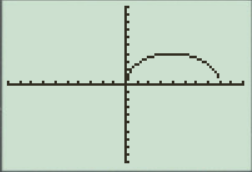Interpretation:

The equation of the function y=8xx2 represents a semicircle.

The equation has a semicircle graph.

The x intercepts are the points on xaxis where the graph of the equation touches xaxis .

The equation of circle is (xa)2+(y1)2=r2 .

The center of circle is either xa=0 or yb=0 .

Thus x=a , y=b .

Thus y is always positive in case of circle then roots give always positive value.

a.

When the x -axis ranges from [4,4] and y -axis ranges from [4,4] their graph obtained in the screen is provided below.

Steps to plot the graph of the equation y=8xx2 with the help of graphing utility are as follows:

Step 1: Press MODE key.

Step 2: Use the down arrow key to reach FUNC option.

Step 3: Press ENTER key.

Step 4: Press Y= key.

Step 5: Enter the function y=8xx2 .

Step 6: Press WINDOW key. Change the settings to

Xmin=4Xmax=4Ymin=4Ymax=4

For better view of graph.

Step 8: Press GRAPH key.

The result obtained on the screen is provided below,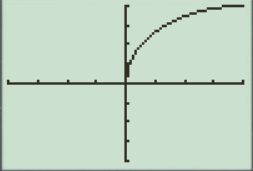This window is not perfect viewing window.

b.

When the x -axis ranges from [5,5] and y -axis ranges from [0,100] their graph obtained in the screen is provided below.

Steps to plot the graph of the equation y=8xx2 with the help of graphing utility are as follows:

Step 1: Press MODE key.

Step 2: Use the down arrow key to reach FUNC option.

Step 3: Press ENTER key.

Step 4: Press Y= key.

Step 5: Enter the function y=8xx2 .

Step 6: Press WINDOW key. Change the settings to

Xmin=5Xmax=5Ymin=0Ymax=100

For better view of graph.

Step 8: Press GRAPH key.

The result obtained on the screen is provided below,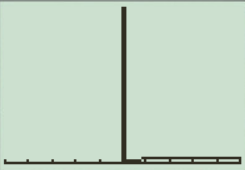This window is not a perfect viewing window.

c.

When the x -axis ranges from [10,10] and y -axis ranges from [10,40] their graph obtained in the screen is provided below.

Steps to plot the graph of the equation y=8xx2 with the help of graphing utility are as follows:

Step 1: Press MODE key.

Step 2: Use the down arrow key to reach FUNC option.

Step 3: Press ENTER key.

Step 4: Press Y= key.

Step 5: Enter the function y=8xx2 .

Step 6: Press WINDOW key. Change the settings to

Xmin=10Xmax=10Ymin=10Ymax=40

For better view of graph.

Step 8: Press GRAPH key.

The result obtained on the screen is provided below,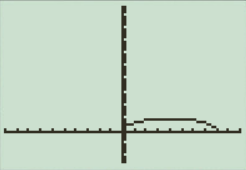This is not the perfect viewing window.

d.

When the x -axis ranges from [2,10] and y -axis ranges from [2,6] their graph obtained in the screen is provided below.

Steps to plot the graph of the equation y=8xx2 with the help of graphing utility are as follows:

Step 1: Press MODE key.

Step 2: Use the down arrow key to reach FUNC option.

Step 3: Press ENTER key.

Step 4: Press Y= key.

Step 5: Enter the function y=8xx2 .

Step 6: Press WINDOW key. Change the settings to

Xmin=2Xmax=10Ymin=2Ymax=6

For better view of graph.

Step 8: Press GRAPH key.

The result obtained on the screen is provided below,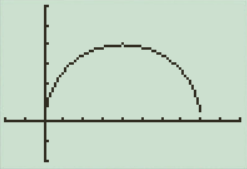This window is the perfect viewing window.

So the perfect viewing window of the graph y=8xx2 is option ‘ d[2,10]by[2,6] .

The perfect viewing window is [2,10]by[2,6] , option ‘ d ’.

### Have a homework question?

Subscribe to bartleby learn! Ask subject matter experts 30 homework questions each month. Plus, you’ll have access to millions of step-by-step textbook answers!